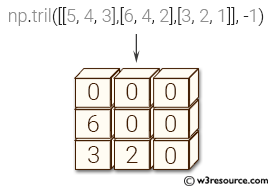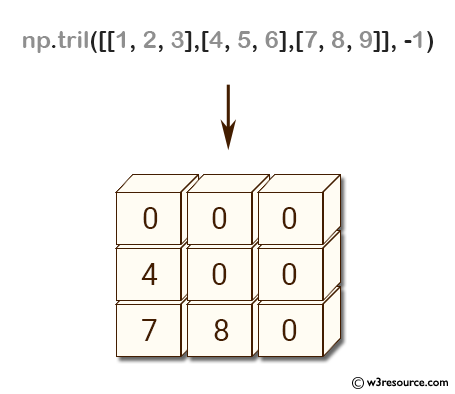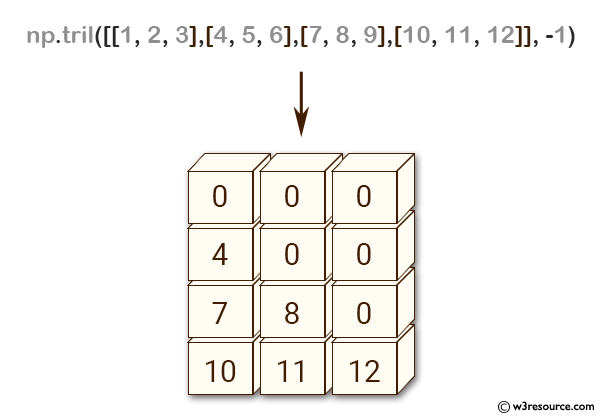# NumPy array creation: tril() function

## numpy.tril() function

The tril() function is used to get a lower triangle of an array.
Return a copy of an array with elements above the k-th diagonal zeroed.

Syntax:

`numpy.tril(m, k=0)`Version: 1.15.0

Parameter:

Name Description Required /
Optional
m Number of rows in the array.
k Diagonal above which to zero elements. k = 0 (the default) is the main diagonal, k < 0 is below it and k > 0 is above. optional

Return value:

tril : ndarray, shape (M, N) - Lower triangle of m, of same shape and data-type as m.

Example-1: NumPy.tril() function

``````>>> import numpy as np
>>> np.tril([[1,2,3],[4,5,6],[7,8,9]], -1)
array([[0, 0, 0],
[4, 0, 0],
[7, 8, 0]])
``````

Pictorial Presentation:Example-2: NumPy.tril() function

``````>>> import numpy as np
>>> np.tril([[1,2,3],[4,5,6],[7,8,9],[10,11,12]], -1)
array([[ 0,  0,  0],
[ 4,  0,  0],
[ 7,  8,  0],
[10, 11, 12]])
``````

Pictorial Presentation:Python - NumPy Code Editor:

Previous: tri()
Next: triu()

﻿